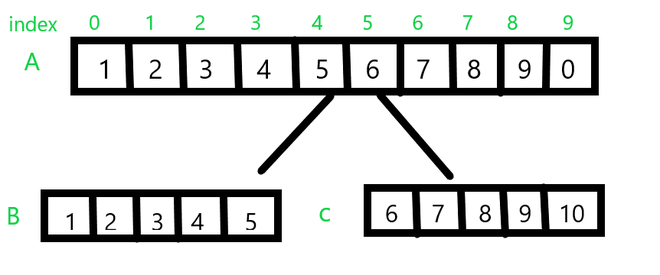# Java Program to Split an Array from Specified Position

• Last Updated : 29 May, 2021

Given an array of size N, our job is to split the array at a specific position specified by the user. Will also discuss the boundary cases.

Consider the following example.From the above example let A be the original array we want to split.  Let N be the length of the array A (N = 10) and let pos be the position we want to split. In the above example pos = 5. All the elements before this position i.e; from index  0 – 4 will be split into one array while elements from index 5 – 10 are split into the later part, labeled as B and C respectively. But if the position is 0 or greater than N then it’s not able to split the array and the invalid position message is displayed.

Examples:

Input: A[] = {1, 2, 3, 4, 5, 6, 7, 8, 9, 0}

pos =  5

Output:  B[] = { 1, 2, 3, 4, 5}

C[] = { 6,7,8,9,0}

Input: A[] = {1, 2, 3, 4, 5, 6, 7, 8, 9, 0}

pos = -1

Output:  Invalid position

Input: A[] = {1, 2, 3, 4, 5, 6, 7, 8, 9, 0}

pos = 20

Output:  Invalid position

Method 1: In the first method, we will be using two for loops. This approach is quite a straight forward method.

• Step 1: first we accept the value of pos from the user
• Step 2: we declare two arrays B and  C having size pos and N – pos respectively.
• Step 3: Then we have two loops the first loop runs from 0 – pos initializing array B while the second loop runs from 0 to N – pos initializing array C.

We have also added a helper method pprint() which accepts an array and prints it.  We also have an if statement checking for valid pos value.

Example:

## Java

 `import` `java.util.*;` `public` `class` `SplittingArray1 {` `    ``// this method accepts a array and prints the value``    ``static` `void` `pprint(``int` `arr[])``    ``{``        ``for` `(``int` `var : arr) {``            ``System.out.print(var + ``" "``);``        ``}` `        ``System.out.println();``    ``}` `    ``public` `static` `void` `main(String args[])``    ``{``        ``// original array``        ``int` `a[] = { ``1``, ``2``, ``3``, ``4``, ``5``, ``6``, ``7``, ``8``, ``9``, ``0` `};` `        ``// size of array``        ``int` `n = a.length;` `        ``// accepting the value of position from the user` `        ``Scanner scanner = ``new` `Scanner(System.in);` `        ``System.out.println(``"Enter position to split."``);` `        ``int` `pos = scanner.nextInt();` `        ``// validating the position for invalid values.``        ``if` `(pos > ``0` `&& pos < n) {``            ``// method  1 : using two for loops` `            ``// declaring array B and C``            ``int` `b[] = ``new` `int``[pos];``            ``int` `c[] = ``new` `int``[n - pos];` `            ``// initializing array B``            ``for` `(``int` `i = ``0``; i < pos; i++) {``                ``b[i] = a[i];``            ``}` `            ``// initializing array C``            ``for` `(``int` `i = ``0``; i < n - pos; i++) {``                ``c[i] = a[i + pos];``            ``}` `            ``// printing the array b and c` `            ``pprint(b);``            ``pprint(c);``        ``}` `        ``else` `{``            ``System.out.println(``"Invalid position."``);``        ``}``    ``}``}`

Output

```Enter position to split.
Invalid position.```

Method 2: In this method instead of using two for loops we try to implement the same program using just one.

• Step 1  and Step 2 are similar to method 1
• Step 3: we run a for loop from  0 to N – 1
``` if index < pos
we initialize array B
else if pos >index
we initialize array C ```

Example:

## Java

 `import` `java.util.Arrays;``import` `java.util.Scanner;` `public` `class` `SplittingArray2 {` `    ``// this method accepts a array and prints the value``    ``static` `void` `pprint(``int` `arr[])``    ``{``        ``for` `(``int` `var : arr) {``            ``System.out.print(var + ``" "``);``        ``}` `        ``System.out.println();``    ``}` `    ``public` `static` `void` `main(String args[])``    ``{` `        ``// original array A``        ``int` `a[] = { ``1``, ``2``, ``3``, ``4``, ``5``, ``6``, ``7``, ``8``, ``9``, ``0` `};` `        ``int` `n = a.length;` `        ``Scanner scanner = ``new` `Scanner(System.in);` `        ``System.out.println(``"Enter position to split."``);` `        ``int` `pos = scanner.nextInt();` `        ``if` `(pos > ``0` `&& pos < n) {``            ``// method  2 : using only one forloop` `            ``int` `b[] = ``new` `int``[pos];``            ``int` `c[] = ``new` `int``[n - pos];` `            ``// only using one for loop to solve the problem.``            ``for` `(``int` `i = ``0``; i < n; i++) {` `                ``if` `(i < pos)``                    ``b[i] = a[i];``                ``else``                    ``c[i - pos] = a[i];``            ``}` `            ``// printing the array b and c` `            ``pprint(b);``            ``pprint(c);``        ``}` `        ``else` `{``            ``System.out.println(``"Invalid position."``);``        ``}``    ``}``}`

Output

```Enter position to split.
Invalid position.```

Method 3:

This is the shortest method. In this method, we use the in-built Arrays.copyOfRange() method.

public static short[] copyOfRange(short[] original, int from, int to)

original − This is the array from which a range is to to be copied.

from − This is the initial index of the range to be copied, inclusive.

to − This is the final index of the range to be copied, exclusive.

Example:

## Java

 `import` `java.util.Arrays;``import` `java.util.Scanner;` `public` `class` `SplittingArray3 {` `    ``static` `void` `pprint(``int` `arr[])``    ``{``        ``for` `(``int` `var : arr) {``            ``System.out.print(var + ``" "``);``        ``}` `        ``System.out.println();``    ``}` `    ``public` `static` `void` `main(String args[])``    ``{``        ``int` `a[] = { ``1``, ``2``, ``3``, ``4``, ``5``, ``6``, ``7``, ``8``, ``9``, ``0` `};` `        ``int` `n = a.length;` `        ``Scanner scanner = ``new` `Scanner(System.in);` `        ``System.out.println(``"Enter position to split."``);` `        ``int` `pos = scanner.nextInt();` `        ``if` `(pos > ``0` `&& pos < n) {` `            ``// method  3 : using  Arrays.copyOfRange()` `            ``int` `b[] = ``new` `int``[pos];``            ``int` `c[] = ``new` `int``[n - pos];` `            ``// initializing array B by copying values from``            ``// index 0  to pos - 1``            ``b = Arrays.copyOfRange(a, ``0``, pos);` `            ``// initializing array B by copying values from``            ``// index pos  to n - 1``            ``c = Arrays.copyOfRange(a, pos, n);` `            ``// printing the array b and c` `            ``pprint(b);``            ``pprint(c);``        ``}` `        ``else` `{``            ``System.out.println(``"Invalid position."``);``        ``}``    ``}``}`

Output

```Enter position to split.
Invalid position.```

Time Complexity: O(n)

Space Complexity: O(n)

My Personal Notes arrow_drop_up